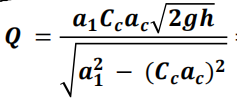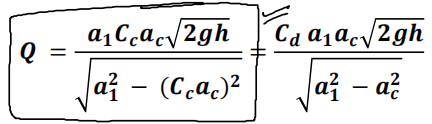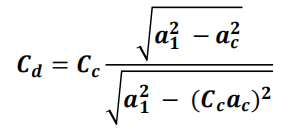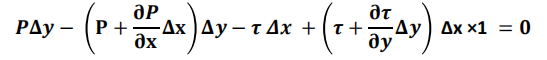# Part #5

## Orifice Meter or Orifice Plate

➢ It is a device used for measuring the rate of flow of a fluid through a pipe.
➢ It is a cheaper device as compared to venturi-meter.
➢ It also works on the same principle as that of venturi-meter.
➢ It consists of a flat circular plate which has a circular sharp edged hole called orifice, which is concentric which concentric with the pipe.
➢ The orifice diameter is kept generally 0.5 times the dimeter of the pipe, though it may vary from 0.4 to 0.8 times the pipe diameter.

### Co-efficient of Contractions

➢ Coefficient of contraction is defined as the proportion between the areas of the stream at the vena contracta to the area of the orifice. It is denoted by the symbol Cc
Cc= a2/ac
➢ Here ac is the area of orifice

### Expression for rate of flow through Orifice-Meter

➢ Similar to venturi-meter by applying Bernoulli's equation we get :### Expression for rate of flow through Orifice-Meter

➢ Equation gives the discharge under ideal conditions and is called, theoretical discharge. Actual discharge will be less than theoretical discharge.➢ By comparing we can see Co-efficient of discharge of orifice meter less than 1. Generally 0.62 to 0.65

## Pitot Tube

➢ It is a device used for measuring the velocity of flow at any point in a pipe or a channel.
➢ It is based on the principle that if the velocity of flow at a point becomes zero, the pressure there is increased due to the conversion of the kinetic energy into pressure energy.
➢ In its simplest form, the pitot-tube consists of a glass tube, bent at right angles as shown in figure.
➢ By applying Bernoulli's Equation we get :
V= √2gh
➢ Equation gives the velocity under ideal conditions and is called, theoretical velocity. Actual velocity will be less than theoretical velocity.
V= Cv√2gh
➢ Here Cv = coefficient of velocity.
➢ The average value of coefficient of velocity for pitot tube is of the order of 0.97.

## Flow of Viscous Fluid Through Circular Pipe

➢ The flow through the circular pipe will be viscous or laminar if the Reynolds number (Re) is less than 2000.
➢ The expression for Reynold number is given by :
Re = ρVd/μ
Where ρ = Density of the fluid
v = average velocity of fluid
D = Diameter of pipe
μ = viscosity of the fluid
➢ Consider a horizontal pipe of radius R. The viscous fluid is flowing from left to right in the pipe as shown in figure.
➢ Consider a fluid element of radius r. Let the length of fluid element be Δx.
➢ Then the forces acting on the fluid element are: R rΔx
➢ The pressure force , p × πr² on front face. P × πr²
➢ The pressure force,(p+dp/dx.Δx) π r² on other face. π r²
➢ The shear force, τ × 2πr Δx on the surface of fluid element
➢ As there is no acceleration, hence the summation of all forces in the direction of flow must be zero i.e.

➢ The shear stress τ across a section varies with ‘r’ as across a section is constant. Hence stress distribution across a section is linear as shown in figure.➢ To obtain the velocity distribution across a section, the value of shear stress τ = μ(du/dy) Is substituted in equation.
➢ The velocity is maximum, when r = 0 in equation. Thus maximum velocity, Umax is obtained as➢ The average velocity, is obtained by dividing the discharge of the fluid across the section by the area of the pipe (πR²).➢ The average velocity, is obtained by dividing the discharge of the fluid across the section by the area of the pipe (πR²)➢ This is called Hagen Poiseuille Formula. Valid only for laminar pipe flow.

## Flow of Viscous Fluid Between Two Parallel Plates

➢ Consider two horizontal parallel plates with separation between them ‘t’ and depth in the plane
is 1 unit. The viscous fluid is flowing from left to right as shown in figure.
➢ Consider a fluid element of thickness Δy. Let the length of fluid element be Δx.
➢ Then the forces acting on the fluid element are:
➢ The pressure force ,( p+ dp/dx) Δy ×1 on front face.
➢ The shear force, τ × (Δx ×1) on the bottom surface of fluid element.
➢ The shear force, (τ+dτ/dy)Δx ×1on the bottom surface of fluid element.
➢ As there is no acceleration, hence the summation of all forces in the direction of flow must be zero i.e.

➢ By solving the equation :➢ Above equation gives Shear Stress at any point.
➢ To obtain the velocity distribution across a section, the value of shear stress τ = μ(du/dy)Is substituted in equation.
➢ The velocity is maximum, when y = t/2 in equation. Thus maximum velocity, Umax is obtained as➢ The average velocity, is obtained by dividing the discharge of the fluid across the section by the area of flow.

## Turbulent Flow Through Circular Pipe

➢ Turbulent flow is that type of flow in which the fluid particles move in a zig-zag way. Due to movement in zig-zag way, the eddies formation takes place which are responsible for high energy loss.
➢ The flow through the circular pipe will be turbulent if the Reynolds number (Re) is greater than 4000.
➢ If 2000 < Re < 4000 flow changes from laminar to turbulent, it is called transition.

### Shear Stress in Turbulent Flow Through Circular Pipe

➢ In turbulent flow, shear stress is develop due to two reasons :
1. Shear stress due to viscosity Ԏv
2. Shear stress due to eddies formation  Ԏe

Ԏ = Ԏv + Ԏe

Ԏ = μ (du/dy) +η (dū/dy)

➢ Here η is called eddy viscosity and its value is very large as compared to viscosity in turbulent flow.
➢ In laminar flow η = 0
➢ Here ū = average velocity up to distance y

## Velocity Distribution in Turbulent Flow Through Circular Pipe

➢ In turbulent flow, Velocity distribution is given by the following relation :

➢ Here Umax is maximum velocity across a section.
➢ u* is called shear velocity.
➢ So it is clear that velocity distribution is logarithmic for turbulent flow through circular pipe.
➢ In laminar flow the difference between average velocity and maximum velocity at a section is large as compared to turbulent flow.

## Kinetic Energy Correction Factors

➢ Kinetic energy correction factor is defined as the ratio of the kinetic energy of the flow per second based on actual velocity across a section to the kinetic energy of the flow per second
based on average velocity across the same section. It is denoted by . Hence Mathematically.

➢ Value of α for laminar flow through pipe is 2.
➢ Value of α for turbulent flow through pipe is 1.03 – 1.06.

## Momentum Correction Factors

➢ Momentum Correction Factor. It is defined as the ratio of momentum of the flow per second
based on actual velocity to the momentum of the flow per second based on average velocity
across a section. It is denoted by β. Hence mathematically.

➢ Value of β for laminar flow through pipe is 1.33.
➢ Value of β for turbulent flow through pipe is 1.015.

## Loss of Energy in Pipes

➢ When a fluid is flowing through a pipe, the fluid experiences some resistance due to which some of the energy of fluid is lost. This loss of energy is classified as:

## Loss of Energy (or Head) Due to Friction

➢ The loss of head (or energy) in pipes due to friction is calculated from Darcy-Weisbach equation which is given by

hf = f.L.V2/2gd

➢ It is valid for both laminar and turbulent flow in pipe.
Here
hf = Loss of head due to friction
f = friction factor = 4 f’ = 4 ( coefficient of friction )
L = length of pipe
V = mean velocity of flow.
d = diameter of pipe.
➢ Friction factor is a function of Reynolds number :
f = 64/Re   for Re < 2000 (laminar flow)
f = 0.316/Re¼  for Re > 4000 (Turbulent flow)

➢ The loss of head or energy due to friction in a pipe is known as major loss while the loss of energy due to change of velocity of the following fluid in magnitude or direction is called minor loss of energy.
➢ The minor loss of energy (or head) includes the following cases :
1. Loss of head due to sudden enlargement,
3. Loss of head at the entrance of a pipe
2. Loss of head due to sudden contraction
5. Loss of head in various pipe fittings
4. Loss of head at the exits of a pipe

### Loss of Head Due to Sudden Enlargement

➢ Consider a liquid flowing through a pipe which has sudden enlargement as shown in figure consider two sections (1)-(1) and (2)-(2) before and after the enlargement.

he = (V12 - V22) /2g

### Loss of Head due to sudden contraction

➢ Consider a liquid flowing in a pipe which has a sudden contraction in area as shown in figure consider two section 1-1 and 2-2 before and after contraction.

hc = V22/2g (1/Cc - 1)2

### Loss of head at the Entrance of a pipe

➢ This is the loss of energy which occurs when a liquid enters a pipe which is connected to a large tank or reservoir.
➢ This loss is similar to the loss of head due to sudden contraction. ➢ This loss depends on the form of entrance.
➢ For a sharp edge entrance, this loss is slightly more than a rounded or bell mouthed entrance.
➢ In practice the value of loss of head at the entrance (or inlet) of a pipe with sharp cornered entrance is taken as

hi =0.5(v2/2g)

where V = velocity of liquid in pipe.

### Loss of head at the Exit of pipe

➢ This is the loss of head (or energy) due to the velocity of liquid at outlet of the pipe which is dissipated either in the form of a free jet (if outlet of the pipe is free) or it is lost in the tank or reservoir (if the outlet of the pipe is connected to the tank or reservoir).
➢ This loss is equal to

ho = V2/2g

➢ Where V = velocity at outlet of pipe.

### Loss of head Due to Bend in Pipe

➢ When there is any bend in a pipe, the velocity of flow changes, due to which the separation of the flow and also formation of eddies takes place.
➢ Thus the energy is lost. Loss of head in pipe due to bend is expressed as

hb = kV2/2g

➢ Where hb = loss of head due to bend, V = Velocity of flow, k = Coefficient of bend The value of k depends on :
➢ Angle of bend, Radius of curvature of bend, Diameter of pipes

### Loss of head in Various Pipe Fittings

➢ The loss of head in the various pipe fittings such as valve, couplings etc, is expressed as

hfitting = kV2/2g

➢ Where V = velocity of flow, k = coefficient of pipe fitting

## Hydraulic Gradient And Total Energy Line

❖ H y d r a u l i c G r a d i e n t L i n e :
It is defined as the line which gives the sum of pressure head and datum head of a flowing fluid in a pipe with respect to some
reference line.
❖ T o t a l E n e r g y l i n e :
It is defined as the line which gives the sum of pressure head, datum head and kinetic head of a flowing fluid in a pipe with respect to some reference line.

THANK YOU VISIT AND SHEAR ....